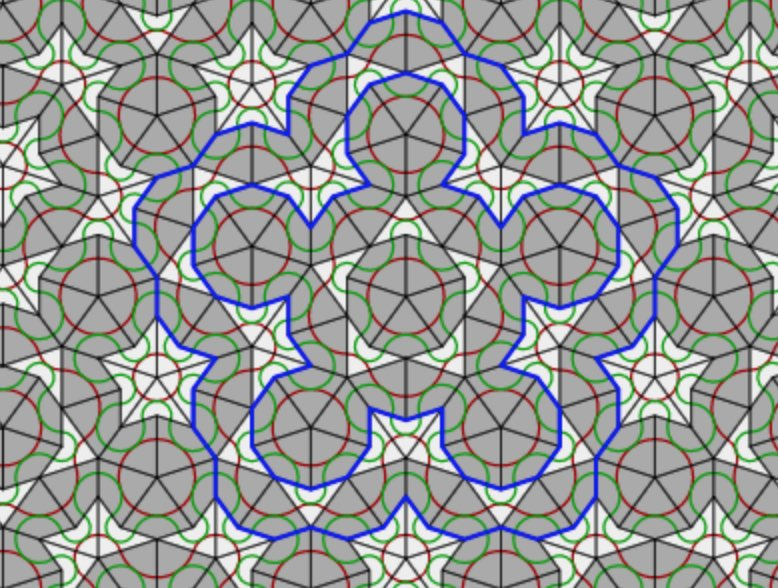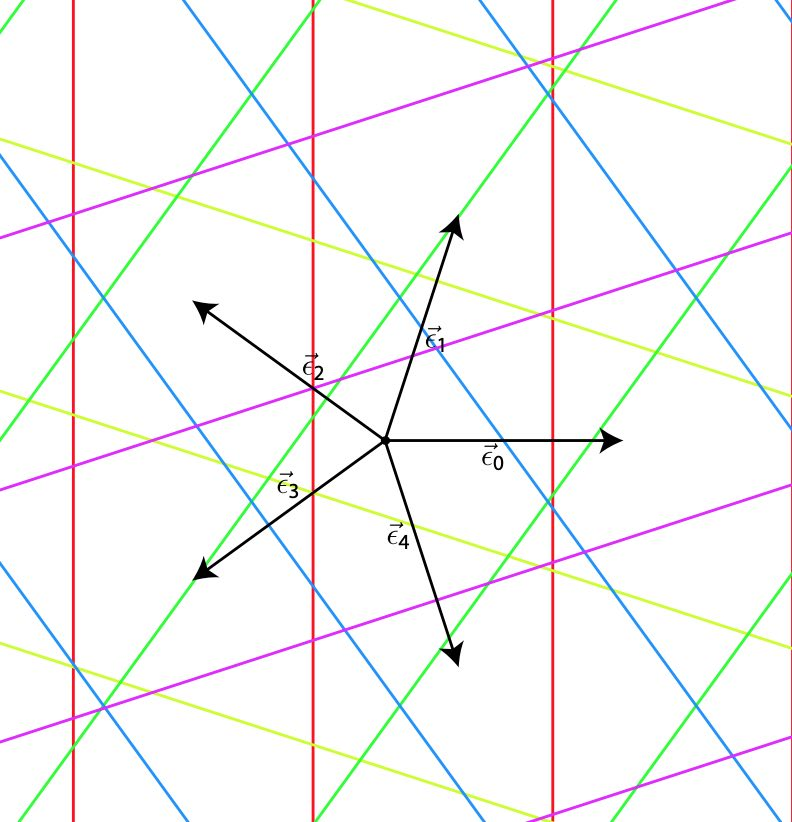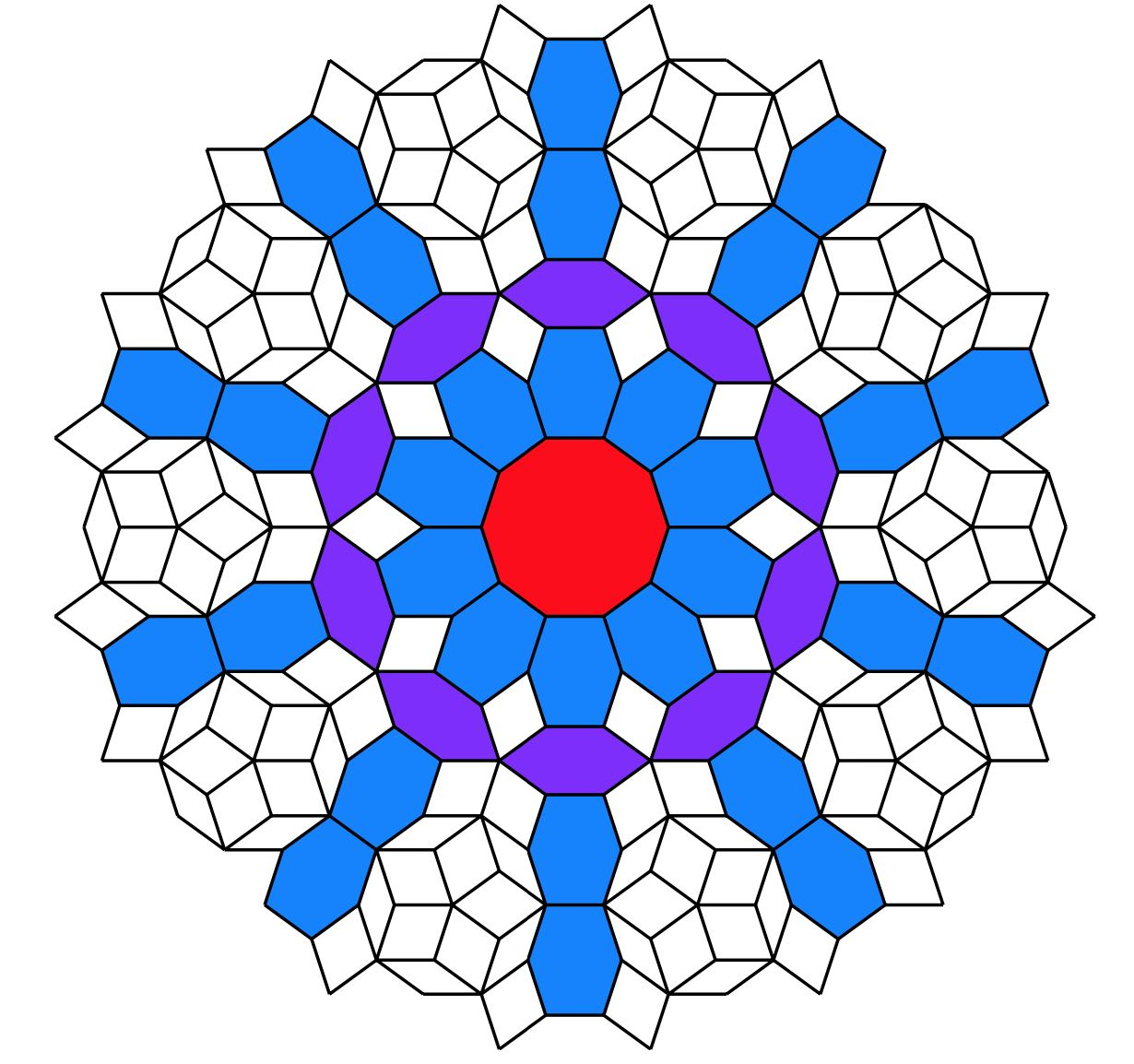Last time we’ve seen that de Bruijn’s pentagrids determined the vertices of Penrose’s P3-aperiodic tilings.

These vertices can also be obtained by projecting a window of the standard hypercubic lattice $\mathbb{Z}^5$ by the cut-and-project-method.

We’ll bring in representation theory by forcing this projection to be compatible with a $D_5$-subgroup of the symmetries of $\mathbb{Z}^5$, which explains why Penrose tilings have a local $D_5$-symmetry.The symmetry group of the standard $n$-dimensional hypercubic lattice
$\mathbb{Z} \vec{e}_1 + \dots + \mathbb{Z} \vec{e}_n \subset \mathbb{R}^n$
is the hyperoctahedral group of all signed $n \times n$ permutation matrices
$B_n = C_2^n \rtimes S_n$
in which all $n$-permutations $S_n$ act on the group $C_2^n = \{ 1,-1 \}^n$ of all signs. The signed permutation $n \times n$ matrix corresponding to an element $(\vec{a},\pi) \in B_n$ is given by
$T_{ij} = T(\vec{a},\pi)_{ij} = a_j \delta_{i,\pi(j)}$
The represenation theory of $B_n$ was worked out in 1930 by the British mathematician and clergyman Alfred YoungWe want to do explicit calculations in $B_n$ using a computational system such as GAP, so it is best to describe $B_n$ as a permutation subgroup of $S_{2n}$ via the morphism
$\tau((\vec{a},\pi))(k) = \begin{cases} \pi(k)+n \delta_{-1,a_k}~\text{if 1 \leq k \leq n} \\ \pi(k-n)+n(1-\delta_{-1,a_{k-n}})~\text{if n+1 \leq k \leq 2n} \end{cases}$
the image is generated by the permutations
$\begin{cases} \alpha = (1,2)(n+1,n+2), \\ \beta=(1,2,\dots,n)(n+1,n+2,\dots,2n), \\ \gamma=(n,2n) \end{cases}$
and to a permutation $\sigma \in \tau(B_n) \subset S_{2n}$ we assign the signed permutation $n \times n$ matrix $T_{\sigma}=T(\tau^{-1}(\pi))$.

We use GAP to set up $B_5$ from these generators and determine all its conjugacy classes of subgroups. It turns out that $B_5$ has no less than $953$ different conjugacy classes of subgroups.

gap> B5:=Group((1,2)(6,7),(1,2,3,4,5)(6,7,8,9,10),(5,10));
Group([ (1,2)(6,7), (1,2,3,4,5)(6,7,8,9,10), (5,10) ])
gap> Size(B5);
3840
gap> C:=ConjugacyClassesSubgroups(B5);;
gap> Length(C);
953

But we are only interested in the subgroups isomorphic to $D_5$. So, first we make a sublist of all conjugacy classes of subgroups of order $10$, and then we go through this list one-by-one and look for an explicit isomorphism between $D_5 = \langle x,y~|~x^5=e=y^2,~xyx=y \rangle$ and a representative of the class (or get a ‘fail’ is this subgroup is not isomorphic to $D_5$).

gap> C10:=Filtered(C,x->Size(Representative(x))=10);;
gap> Length(C10);
3
gap> s10:=List(C10,Representative);
[ Group([ (2,5)(3,4)(7,10)(8,9), (1,5,4,3,2)(6,10,9,8,7) ]),
Group([ (1,6)(2,5)(3,4)(7,10)(8,9), (1,10,9,3,2)(4,8,7,6,5) ]),
Group([ (1,6)(2,7)(3,8)(4,9)(5,10), (1,2,8,4,10)(3,9,5,6,7) ]) ]
gap> D:=DihedralGroup(10); gap> IsomorphismGroups(D,s10);
[ f1, f2 ] -> [ (2,5)(3,4)(7,10)(8,9), (1,5,4,3,2)(6,10,9,8,7) ]
gap> IsomorphismGroups(D,s10);
[ f1, f2 ] -> [ (1,6)(2,5)(3,4)(7,10)(8,9), (1,10,9,3,2)(4,8,7,6,5) ]
gap> IsomorphismGroups(D,s10);
fail
gap> IsCyclic(s10);
true

Of the three (conjugacy classes of) subgroups of order $10$, two are isomorphic to $D_5$, and the third one to $C_{10}$. Next, we have to transform the generating permutations into signed $5 \times 5$ permutation matrices using the bijection $\tau^{-1}$.
$\begin{array}{c|c} \sigma & (\vec{a},\pi) \\ \hline (2,5)(3,4)(7,10)(8,9) & ((1,1,1,1,1),(2,5)(3,4)) \\ (1,5,4,3,2)(6,10,9,8,7) & ((1,1,1,1,1)(1,5,4,3,2)) \\ (1,6)(2,5)(3,4)(7,10)(8,9) & ((-1,1,1,1,1),(2,5)(3,4)) \\ (1,10,9,3,2)(4,8,7,6,5) & ((-1,1,1,-1,1),(1,5,4,3,2)) \end{array}$
giving the signed permutation matrices
$\begin{array}{c|cc} & x & y \\ \hline A & \begin{bmatrix} 0 & 1 & 0 & 0 & 0 \\ 0 & 0 & 1 & 0 & 0 \\ 0 & 0 & 0 & 1 & 0 \\ 0 & 0 & 0 & 0 & 1 \\ 1 & 0 & 0 & 0 & 0 \end{bmatrix} & \begin{bmatrix} 1 & 0 & 0 & 0 & 0 \\ 0 & 0 & 0 & 0 & 1 \\ 0 & 0 & 0 & 1 & 0 \\ 0 & 0 & 1 & 0 & 0 \\ 0 & 1 & 0 & 0 & 0 \end{bmatrix} \\ \hline B & \begin{bmatrix} 0 & 1 & 0 & 0 & 0 \\ 0 & 0 & 1 & 0 & 0 \\ 0 & 0 & 0 & -1 & 0 \\ 0 & 0 & 0 & 0 & 1 \\ -1 & 0 & 0 & 0 & 0 \end{bmatrix} & \begin{bmatrix} -1 & 0 & 0 & 0 & 0 \\ 0 & 0 & 0 & 0 & 1 \\ 0 & 0 & 0 & 1 & 0 \\ 0 & 0 & 1 & 0 & 0 \\ 0 & 1 & 0 & 0 & 0 \end{bmatrix} \end{array}$
$D_5$ has $4$ conjugacy classes with representatives $e,y,x$ and $x^2$. the
character table of $D_5$ is
$\begin{array}{c|cccc} & (1) & (2) & (2) & (5) \\ & 1_a & 5_1 & 5_2 & 2_a \\ D_5 & e & x & x^2 & y \\ \hline T & 1 & 1 & 1 & 1 \\ V & 1 & 1 & 1 & -1 \\ W_1 & 2 & \tfrac{-1+ \sqrt{5}}{2} & \tfrac{-1 -\sqrt{5}}{2} & 0 \\ W_2 & 2 & \tfrac{-1 -\sqrt{5}}{2} & \tfrac{-1+\sqrt{5}}{2} & 0 \end{array}$
Using the signed permutation matrices it is easy to determine the characters of the $5$-dimensional representations $A$ and $B$
$\begin{array}{c|cccc} D_5 & e & x & x^2 & y \\ \hline A & 5 & 0 & 0 & 1 \\ B & 5 & 0 & 0 & -1 \end{array}$
decomosing into $D_5$-irreducibles as
$A \simeq T \oplus W_1 \oplus W_2 \quad \text{and} \quad B \simeq V \oplus W_1 \oplus W_2$
Representation $A$ realises $D_5$ as a rotation symmetry group of the hypercube lattice $\mathbb{Z}^5$ in $\mathbb{R}^5$, and next we have to find a $D_5$-projection $\mathbb{R}^5=A \rightarrow W_1 = \mathbb{R}^2$.

As a complex representation $A \downarrow_{C_5}$ decomposes as a direct sum of $1$-dimensional representations
$A \downarrow_{C_5} = V_1 \oplus V_{\zeta} \oplus V_{\zeta^2} \oplus V_{\zeta^3} \oplus V_{\zeta^4}$
where $\zeta = e^{2 \pi i /5}$ and where the action of $x$ on $V_{\zeta^i}=\mathbb{C} v_i$ is given by $x.v_i = \zeta^i v_i$. The $x$-eigenvectors in $\mathbb{C}^5$ are
$\begin{cases} v_0 = (1,1,1,1,1) \\ v_1 = (1,\zeta,\zeta^2,\zeta^3,\zeta^4) \\ v_2 =(1,\zeta^2,\zeta^4,\zeta,\zeta^3) \\ v_3 = (1,\zeta^3,\zeta,\zeta^4,\zeta^2) \\ v_4 = (1,\zeta^4,\zeta^3,\zeta^2,\zeta) \end{cases}$
The action of $y$ on these vectors is given by $y.v_i = v_{5-i}$ because
$x.(y.v_i) = (xy).v_i=(yx^{-1}).v_i=y.(x^{-1}.v_i) = y.(\zeta^{-i} v_i) = \zeta^{-1} (y.v_i)$
and therefore $y.v_i$ is an $x$-eigenvector with eigenvalue $\zeta^{5-i}$. As a complex $D_5$-representation, the factors of $A$ are therefore
$T = \mathbb{C} v_0, \quad W_1 = \mathbb{C} v_1 + \mathbb{C} v_4, \quad \text{and} \quad W_2 = \mathbb{C} v_2 + \mathbb{C} v_3$
But we want to consider $A$ as a real representation. As $\zeta^j = cos(\tfrac{2 \pi j}{5})+i~sin(\tfrac{2 \pi j}{5}) = c_j + i s_j$ hebben we can take the vectors in $\mathbb{R}^5$
$\begin{cases} \frac{1}{2}(v_1+v_4) = (1,c_1,c_2,c_3,c_4)= u_1 \\ -\frac{1}{2}i(v_1-v_4) = (0,s_1,s_2,s_3,s_4) = u_2 \\ \frac{1}{2}(v_2+v_3) = (1,c_2,c_4,c1,c3)= w_1 \\ -\frac{1}{2}i(v_2-v_3) = (0,s_2,s_4,s_1,s_3)= w_2 \end{cases}$
and $A$ decomposes as a real $D_5$-representation with
$T = \mathbb{R} v_0, \quad W_1 = \mathbb{R} u_1 + \mathbb{R} u_2, \quad \text{and} \quad W_2 = \mathbb{R} w_1 + \mathbb{R} w_2$
and if we identify $\mathbb{C}$ with $\mathbb{R}^2$ via $z \leftrightarrow (Re(z),Im(z))$ we can describe the $D_5$-projection morphism $\pi_{W_1}~:~\mathbb{R}^5=A \rightarrow W_1=\mathbb{R}^2$ via
$(y_0,y_1,y_2,y_3,y_4) \mapsto y_0+y_1 \zeta + y_2 \zeta^2 + y_3 \zeta^3 + y_4 \zeta^4 = \sum_{i=0}^4 y_i (c_i,s_i)$
Note also that $W_1$ is the orthogonal complement of $T \oplus W_2$, so is equal to the linear subspace in $\mathbb{R}^5$ determined by the three linear equations
$\begin{cases} \sum_{i=0}^4 x_i = 0 \\ \sum_{i=0}^4 c_{2i} x_i = 0 \\ \sum_{i=0}^4 s_{2i} x_i = 0 \end{cases}$Okay, now take the Rhombic tiling corresponding to the regular pentagrid defined by $\gamma_0, \dots, \gamma_4$ satisfying $\sum_{i=0}^4 \gamma_i = 0$. Let $\vec{k}=(k_0,\dots,k_4) \in \mathbb{Z}^5$ and define the open hypercube $H_{\vec{k}}$ corresponding to $\vec{k}$ as the set of points
$(x_0,\dots,x_4) \in \mathbb{R}^5~:~\forall 0 \leq i \leq 4~:~k_i – 1 < x_i < k_i$ From the vector $\vec{\gamma} = (\gamma_0,\dots,\gamma_4)$ determining the Rhombic tiling we define the $2$-dimensional plane $P_{\vec{\gamma}}$ in $\mathbb{R}^5$ given by the equations $\begin{cases} \sum_{i=0}^4 x_i = 0 \\ \sum_{i=0}^4 c_{2i} (x_i - \gamma_i) = 0 \\ \sum_{i=0}^4 s_{2i} (x_i - \gamma_i) = 0 \end{cases}$ The point being that $P_{\vec{\gamma}}$ is the linear plane $W_1$ in $\mathbb{R}^5$ translated over the vector $\vec{\gamma}$, so it is parallel to $W_1$. Here's the punchline:

de Bruijn’s theorem: The vertices of the Rhombic tiling produced by the regular pentagrid with parameters $\vec{\gamma}=(\gamma_0,\dots,\gamma_4)$ are the points
$\sum_{i=0}^4 k_i (c_i,s_i)$
with $\vec{k}=(k_0,\dots,k_4) \in \mathbb{Z}^5$ such that $H_{\vec{k}} \cap P_{\vec{\gamma}} \not= \emptyset$.

To see this, let $\vec{x} = (x_0,\dots,x_4) \in P_{\vec{\gamma}}$, then $\vec{x}-\vec{\gamma} \in W_1$, but then there is a vector $\vec{y} \in \mathbb{R}^2$ such that
$x_j – \gamma_j = \vec{y}.\vec{v}_j \quad \forall~0 \leq j \leq 4$
But then, with $k_j=\lceil \vec{y}.\vec{v}_j + \gamma_j \rceil$ we have that $\vec{x} \in H_{\vec{k}}$ and we note that $V(\vec{y}) = \sum_{i=0}^4 k_i \vec{v}_i$ is a vetex of the Rhombic tiling associated to the regular pentagrid parameters $\vec{\gamma}=(\gamma_0,\dots,\gamma_4)$.

Here we used regularity of the pentagrid in order to have that $k_j=\vec{y}.\vec{v}_j + \gamma_j$ can happen for at most two $j$’s, so we can manage to vary $\vec{y}$ a little in order to have $\vec{x}$ in the open hypercube.

Here’s what we did so far: we have identified $D_5$ as a group of rotations in $\mathbb{R}^5$, preserving the hypercube-lattice $\mathbb{Z}^5$ in $\mathbb{R}^5$. If the $2$-plane $P_{\vec{\gamma}}$ is left stable under these rotations, then because rotations preserve distances, also the subset of lattice-points
$S_{\vec{\gamma}} = \{ (k_0,\dots,k_4)~|~H_{\vec{k}} \cap P_{\vec{\gamma}} \not= \emptyset \} \subset \mathbb{Z}^5$
is left stable under the $D_5$-action. But, because the map
$(k_0,\dots,k_4) \mapsto \sum_{i=0}^4 k_i (c_i,s_i)$
is the $D_5$-projection map $\pi : A \rightarrow W_1$, the vertices of the associated Rhombic tiling must be stable under the $D_5$-action on $W_1$, meaning that the Rhombic tiling should have a global $D_5$-symmetry.

Sadly, the only plane $P_{\vec{\gamma}}$ left stable under all rotations of $D_5$ is when $\vec{\gamma} = \vec{0}$, which is an exceptionally singular pentagrid. If we project this situation we do indeed get an image with global $D_5$-symmetrybut it is not a Rhombic tiling. What’s going on?

Because this post is already dragging on for far too long (TL;DR), we’ll save the investigation of projections of singular pentagrids, how they differ from the regular situation, and how they determine multiple Rhombic tilings, for another time.

Published in geometry GoV groups representations